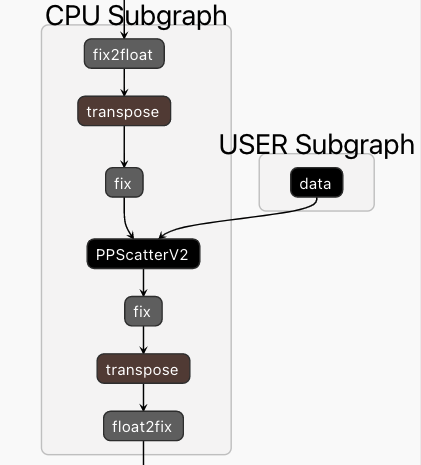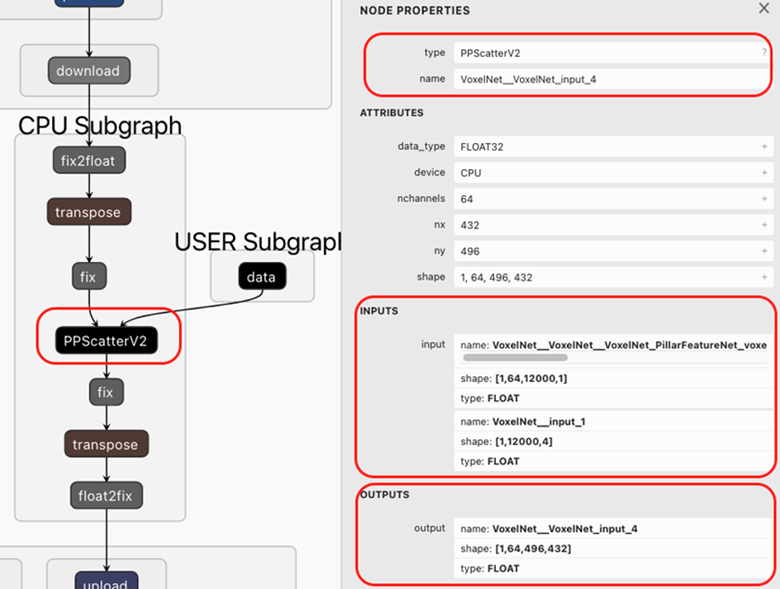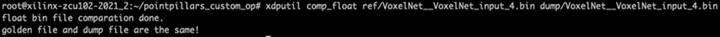# Custom OP Registration - 2.0 English

## Vitis AI User Guide (UG1414)

Document ID
UG1414
Release Date
2022-01-20
Version
2.0 English

Before custom op registration, you can use the latest Netron program to check the compiled model, as shown below. From the graph displayed, PPScatter is assigned to the CPU and we have to implement and register PPScatter OP.

Figure 1. PPScatter OP in CPU Subgraph## Steps

1. Use Netron to open the compiled model, and find the custom OP in CPU subgraph with op information.
Figure 2. The inputs and outputs of PPScatter OpFrom the above model structure image, you can find the OP type is PPScatterV2 which is the name of the custom OP that needs to be created.

2. Write your own implementation of this op.

There is README.md file in Vitis-AI/demo/Custom_OP_Demo/op_add directory which illustrates the detailed steps on how to implement your own op. Please study that detailed document carefully.

Below is an example on how to implement the PPScatterV2 op.

1. Create the my_PPScatter_op.cpp source file and put it under new folder op_PPScatter.

You can also copy one existed op and renamed to your op, as shown below. Then, rename my_tanh_op.cpp to my_PPScatter_op.cpp.

``cp - r Vitis-AI/tools/Vitis-AI-Library/cpu_task/examples/op_tanh/  op_PPScatter ``
2. Create the Makefile.
The Makefile is shown below.
``````
OUTPUT_DIR = \$(HOME)/build/customer_op

all: \$(OUTPUT_DIR) \$(OUTPUT_DIR)/libvart_op_imp_PPScatterV2.so

\$(OUTPUT_DIR):
mkdir -p \$@

\$(OUTPUT_DIR)/my_PPScatter_op.o: my_PPScatter_op.cpp
\$(CXX) -std=c++17 -fPIC -c -o \$@ -I. -I=/install/Debug/include -Wall -U_FORTIFY_SOURCE -D_FORTIFY_SOURCE=0 \$<

\$(OUTPUT_DIR)/libvart_op_imp_PPScatterV2.so: \$(OUTPUT_DIR)/my_PPScatter_op.o
\$(CXX) -Wl,--no-undefined -shared -o \$@ \$+ -L=/install/Debug/lib -lglog -lvitis_ai_library-runner_helper -lvart-runner -lxir``````
3. Write the implementation of the op.

In my_PPScatter_op.cpp, use the construct function to initialize some variable; in this example, there is no variable need be initialized.

In the calculate() function, implementation your own logic. The logic is mainly getting input data from “inputs” variable, calculating the logic, writing output data to the “output” variable.

The code of my_PPScatter_op.cpp is shown below.
``````
#include <vart/op_imp.h>

class MyPPScatterOp {
public:
MyPPScatterOp(const xir::Op* op1, xir::Attrs* attrs) : op{op1} {
// op and attrs is not in use.
}

int calculate(vart::simple_tensor_buffer_t output,
std::vector<vart::simple_tensor_buffer_t<float>> inputs) {
CHECK_EQ(inputs.size(), 2);
auto input_data_shape = inputs.tensor->get_shape();
auto input_coord_shape = inputs.tensor->get_shape();
auto output_shape = output.tensor->get_shape();
CHECK_EQ(input_data_shape.size(), 4); // 1 12000 1 64 --> 1 64 12000 1
CHECK_EQ(input_coord_shape.size(), 3); // 1 12000 4
CHECK_EQ(output_shape.size(), 4); // 1 496 432 64 ---> 1 64 496 432

auto coord_numbers = input_coord_shape;
auto coord_channel = input_coord_shape;
CHECK_EQ(coord_numbers, input_data_shape);

auto batch = output_shape;
auto height = output_shape;
auto width = output_shape;
auto channel = output_shape;
CHECK_EQ(input_data_shape, batch);
CHECK_EQ(channel, input_data_shape);

auto output_idx = 0;
auto input_idx = 0;
auto x_idx = 0;

memset(output.data, 0, output_shape*output_shape*output_shape*output_shape*sizeof(float));

for (auto n = 0; n < coord_numbers; n++) {
auto x = (int)inputs.data[x_idx + 3];
auto y = (int)inputs.data[x_idx + 2];
if (x < 0) break; // stop copy data when coord x == -1 .
for(int i=0; i < channel; i++) {
output_idx =i*height*width + y*width+x;
input_idx = n+i*coord_numbers;
output.data[output_idx] = inputs.data[ input_idx ];
}
x_idx += coord_channel;
}
return 0;
}

public:
const xir::Op* const op;
};

DEF_XIR_OP_IMP(MyPPScatterOp)
``````
4. Build the library. The target directory is \$(HOME)/build/custom_op/ .  You can modify the path in Makefile.

Running make with your Makefile, you’ll see the custom defined op library is generated in \$(HOME)/build/custom_op/, file name is libvart_op_imp_PPScatterV2.so.

5. Copy the libvart_op_imp_PPScatterV2.so to /usr/lib on the target.
3. Verify the Op on the target.
1. Use run_op command in xdputil to test the op, as shown below.
``xdputil run_op pointpillars_op.xmodel VoxelNet__VoxelNet_input_4 -r ref -d dump``

Before running the above command, prepare the reference inputs of the op. After you run the command successfully, VoxelNet__VoxelNet_input_4.bin file will be generated.

2. Comparing the output with the golden file. The command is shown below.
`` xdputil comp_float ref/VoxelNet__VoxelNet_input_4.bin dump/VoxelNet__VoxelNet_input_4.bin``

The following result shows the OP you implemented is correct.